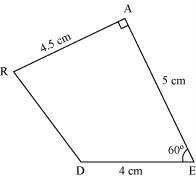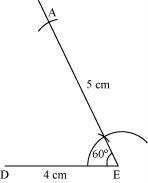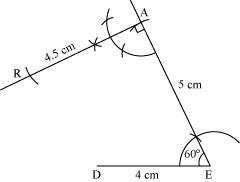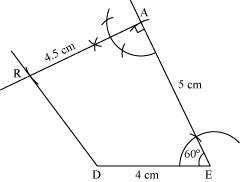# Construct the following quadrilateral: Quadrilateral DEAR DE = 4 cm EA = 5 cm AR = 4.5 cm ∠E = 60° ∠A = 90°

SolutionWe can draw the quadrilateral DEAR using the steps given below

(1) Draw a line segment DE of 4 cm and an angle of 60º at point E. As vertex A is 5 cm away from vertex E, cut a line segment EA of 5 cm from this ray.(2) Again draw an angle of 90º at point A. As vertex R is 4.5 cm away from vertex A, cut a line segment RA of 4.5 cm from this ray.(3) Join D to R.(0)(0)